﻿ 一个有任意给定数目平衡点的四维超混沌系统

一个有任意给定数目平衡点的四维超混沌系统A 4D Hyperchaotic System with Any Given Number of Equilibria

Abstract: This paper introduces a 4D hyperchaotic ODE system based on segmented disc dynamo system. The system can have any given number of equilibrium when parameters vary. Many interesting chaotic properties of the system are also given: (i) a hidden chaotic attractor exists with no equilibria; (ii) a hyperchaotic attractor exists with two non-hyperbolic equilibria; (iii) a chaotic attractor exists with six unstable equilibria; (iv) chaotic attractors coexist with infinitely many unstable isolated equilibria; (v) a chaotic attractor exists with line equilibrium. The paper further proves that Hopf bifurcation occurs simultaneously at two equilibria in the system under appropriate parameter conditions. Numerical simulation demonstrates the emergence of the Hopf bifurcation.

1. 引言

2. 新的四维超混沌系统

2.1. 系统形式

Moffatt  提出了一种分段盘式发电机模型如下：

$\left\{\begin{array}{l}\stackrel{˙}{x}=r\left(y-x\right),\\ \stackrel{˙}{y}=mx-\left(1+m\right)y+xz,\\ \stackrel{˙}{z}=g\left(m{x}^{2}+1-\left(1+m\right)xy\right).\end{array}$

$\left\{\begin{array}{l}\stackrel{˙}{x}=r\left(y-x\right),\\ \stackrel{˙}{y}=mx-\left(1+m\right)y+xz,\\ \stackrel{˙}{z}=g\left(m{x}^{2}+1-\left(1+m\right)xy\right).\end{array}$ (1)

${S}_{0}=\left\{\begin{array}{c}\left(r,m,g,{k}_{1},{k}_{2},{k}_{3},{k}_{4},{k}_{5},{k}_{7}\right)|\begin{array}{c}r,m,g>0,{k}_{5}\ge 0,{k}_{2}\le 0,\\ g-{k}_{3}\ne 0,|{k}_{1}|<1+m+r+{k}_{5}\end{array}\end{array}\right\}.$

$\text{div}\text{ }F=\frac{\partial {F}_{1}}{\partial x}+\frac{\partial {F}_{2}}{\partial y}+\frac{\partial {F}_{3}}{\partial z}+\frac{\partial {F}_{4}}{\partial u}=-\left(r+1+m+{k}_{5}+{k}_{1}\mathrm{cos}u\right)<0.$

$\stackrel{\to }{p}=\left(0.1,100,0.1,0,-1,1,-1,0,1\right)$，此时系统(1)没有平衡点。选取初始条件为 $\left(0,0,0,0\right)$，则得到系统(1)有一个隐藏超混沌吸引子，四个李雅普诺夫指数为

$\left({\lambda }_{LE1},{\lambda }_{LE2},{\lambda }_{LE3},{\lambda }_{LE4}\right)=\left(0.1904,0.1877,0.0000,-17.6277\right),$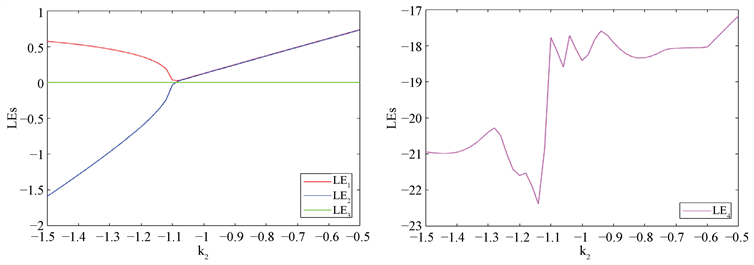Figure 1. Parameters $\left(r,m,g,{k}_{1},{k}_{3},{k}_{4},{k}_{5},{k}_{7}\right)=\left(0.1,100,0.1,0,1,-1,0,1\right)$, the initial condition is $\left(0,0,0,0\right)$, ${k}_{2}\in \left[-1.5,-0.5\right]$ Lyapunov exponent spectrum of system (1)

2.2. 平衡点的数量

(I)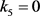的情形

(1) 令 ${k}_{1}=0$ 并且 ${k}_{7}\ne 0$，则

(a) 如果 $\left({k}_{2}+g-{k}_{4}\right)\left(g-{k}_{3}\right)<0$，则系统(1)没有平衡点；

(b) 如果 ${k}_{2}+g-{k}_{4}=0$，则系统(1)有一条线平衡点

${E}^{\text{*}}\left(0,0,{z}^{\text{*}},0\right),{z}^{\text{*}}\in ℝ\text{.}$

(c) 如果 $\left({k}_{2}+g-{k}_{4}\right)\left(g-{k}_{3}\right)>0$，则系统(1)有两个平衡点

${E}_{±}\left(±{x}_{0},±{x}_{0},1,0\right),$

${x}_{0}=\sqrt{\frac{{k}_{2}+g-{k}_{4}}{g-{k}_{3}}}.$

(2) 令 ${k}_{1}{k}_{2}\ne 0$${k}_{7}=0$ 并且 $|g-{k}_{4}|\le |{k}_{2}|$，则系统(1)有无穷多个孤立平衡点，具体如下：

(a) 如果 ${k}_{2}\left(g-{k}_{3}\right)>0$，则系统(1)的平衡点为

${E}_{±,k}\left(±{x}^{*},±{x}^{*},1,2k\pi \right),k\in ℤ\text{.}$

(b) 如果 ${k}_{2}\left(g-{k}_{3}\right)<0$，则系统(1)的平衡点为

${E}_{±,k}\left(±{x}^{*},±{x}^{*},1,\left(2k+1\right)\pi \right),k\in ℤ\text{.}$

${x}^{*}=\sqrt{\frac{|{k}_{2}|\mathrm{sgn}\left(g-{k}_{3}\right)+g-{k}_{4}}{g-{k}_{3}}}\text{.}$

(3) 令 ${k}_{1}{k}_{7}\ne 0$ 并且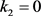，则

(a) 如果 $\left(g-{k}_{3}\right)\left(g-{k}_{4}\right)<0$，则系统(1)没有平衡点；

(b) 如果 $g-{k}_{4}=0$，则系统(1)有一条线平衡点

${E}^{\text{*}}\left(0,0,{z}^{*},0\right),{z}^{*}\in ℝ\text{.}$

(c) 如果 $\left(g-{k}_{3}\right)\left(g-{k}_{4}\right)>0$，则系统(1)有 $2\left(2N+1\right)$ 个平衡点，其中N可以是任意预先给定非负整数。

(4) 令 ${k}_{1}{k}_{2}{k}_{7}\ne 0$ 并且 $g-{k}_{3}>0$，则系统(1)有2N个平衡点，其中N可以是任意预先给定非负整数。

(II) ${k}_{5}>0$ 并且 $g-{k}_{3}>0$ 的情形

(1) 令 ${k}_{1}=0$${k}_{2}{k}_{7}\ne 0$ 并且 $-{k}_{5}+{k}_{2}+g-{k}_{4}\le 0$，则系统(1)有唯一平衡点

${E}_{0}\left(0,0,\frac{{k}_{2}+g-{k}_{4}}{{k}_{5}},0\right)\text{.}$

(2) 令 ${k}_{1}{k}_{2}\ne 0$${k}_{7}=0$ 并且 $-{k}_{2}+g-{k}_{4}\le 0$，则系统(1)有无穷多个孤立平衡点

${E}_{1,k}\left(0,0,\frac{{k}_{2}+g-{k}_{4}}{{k}_{5}},2k\pi \right),k\in ℤ,$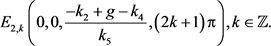(3) 令 ${k}_{1}{k}_{7}\ne 0$${k}_{2}=0$ 并且 $g-{k}_{4}\le {k}_{5}$，则系统(1)可以有任意给定奇数个平衡点。

$\left\{\begin{array}{l}{F}_{2}\left({E}_{0}\right)={x}_{0}\left({z}_{0}-1\right)-{k}_{7}{u}_{0}=0,\\ {F}_{3}\left({E}_{0}\right)=-\left(g-{k}_{3}\right){x}_{0}^{2}+\left(-{k}_{5}{z}_{0}+{k}_{2}\mathrm{cos}{u}_{0}+g-{k}_{4}\right)=0,\\ {F}_{4}\left({E}_{0}\right)={x}_{0}\left({z}_{0}-1\right)-{k}_{1}\mathrm{sin}{u}_{0}=0.\end{array}$ (2)

(I) ${k}_{5}=0$ 的情形

(1) 当 ${k}_{1}=0$ 并且 ${k}_{7}\ne 0$ 时，由 ${F}_{2}\left({E}_{0}\right)={F}_{4}\left({E}_{0}\right)=0$ 可得 ${u}_{0}=0$。于是

${F}_{3}\left({E}_{0}\right)=-\left(g-{k}_{3}\right){x}_{0}^{2}+\left({k}_{2}+g-{k}_{4}\right).$

(a) 如果 $\left({k}_{2}+g-{k}_{4}\right)\left(g-{k}_{3}\right)<0$，则系统(1)没有平衡点；

(b) 如果 ${k}_{2}+g-{k}_{4}=0$，由 ${F}_{3}\left({E}_{0}\right)=0$ 可得 ${x}_{0}=0$，这直接可以推得 ${F}_{4}\left({E}_{0}\right)=0$。因此 ${z}_{0}\in ℝ$

(c) 如果 $\left({k}_{2}+g-{k}_{4}\right)\left(g-{k}_{3}\right)>0$，由 ${F}_{3}\left({E}_{0}\right)=0$ 可得

${x}_{0}=±\sqrt{\frac{{k}_{2}+g-{k}_{4}}{g-{k}_{3}}}\text{.}$

(2) 当 ${k}_{1}{k}_{2}\ne 0$${k}_{7}=0$ 并且 $|g-{k}_{4}|\le |{k}_{2}|$ 时，由 ${F}_{2}\left({E}_{0}\right)={F}_{4}\left({E}_{0}\right)=0$ 可得 ${u}_{0}=k\pi$，因此 $\mathrm{cos}{u}_{0}=±1$。很显然，

$\mathrm{sgn}\left({k}_{2}\mathrm{cos}{u}_{0}+g-{k}_{4}\right)=\mathrm{sgn}\left({k}_{2}\mathrm{cos}{u}_{0}\right)\ne 0.$

$\mathrm{sgn}\left(g-{k}_{3}\right)=\mathrm{sgn}\left({k}_{2}\mathrm{cos}{u}_{0}+g-{k}_{4}\right)=\mathrm{sgn}\left({k}_{2}\mathrm{cos}{u}_{0}\right).$

${x}^{*}=\sqrt{\frac{|{k}_{2}|\mathrm{sgn}\left(g-{k}_{3}\right)+g-{k}_{4}}{g-{k}_{3}}}\text{.}$

(a) 如果 ${k}_{2}\left(g-{k}_{3}\right)>0$，则系统(1)的平衡点为

${E}_{±,k}\left(±{x}^{*},±{x}^{*},1,2k\pi \right),k\in ℤ\text{.}$

(b) 如果 ${k}_{2}\left(g-{k}_{3}\right)<0$，则系统(1)的平衡点为

${E}_{±,k}\left(±{x}^{*},±{x}^{*},1,\left(2k+1\right)\pi \right),k\in ℤ\text{.}$

(3) 当 ${k}_{1}{k}_{7}\ne 0$ 并且 ${k}_{2}=0$ 时，有

${F}_{3}\left({E}_{0}\right)=-\left(g-{k}_{3}\right){x}_{0}^{2}+\left(g-{k}_{4}\right).$

${k}_{7}{u}_{0}={k}_{1}\mathrm{sin}{u}_{0},$

${E}_{±}\left(±{x}^{*},±{x}^{*},1,0\right),$

${P}_{±}^{\left(n\right)}\left(±{x}^{*},±{x}^{*},\frac{±{x}^{*}+{k}_{7}{u}_{n}}{±{x}^{*}},{u}_{n}\right),n=1,2,\cdots ,N,$

${Q}_{±}^{\left(n\right)}\left(±{x}^{*},±{x}^{*},\frac{±{x}^{*}-{k}_{7}{u}_{n}}{±{x}^{*}},-{u}_{n}\right),n=1,2,\cdots ,N.$

${x}^{*}=\sqrt{\frac{g-{k}_{4}}{g-{k}_{3}}}.$

(4) 当 ${k}_{1}{k}_{2}{k}_{7}\ne 0$ 并且 $g-{k}_{3}>0$ 时，仍设关于 ${u}_{0}$ 的方程 ${k}_{7}{u}_{0}={k}_{1}\mathrm{sin}{u}_{0}$$2N+1$ 个解 $0,±{u}_{1},±{u}_{2},\cdots ,±{u}_{N}$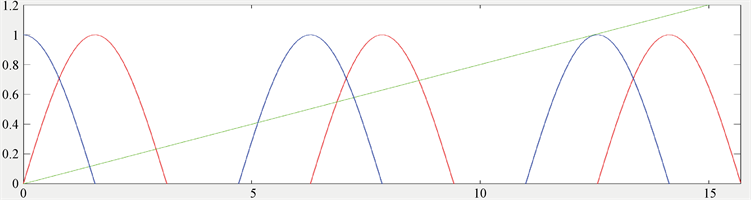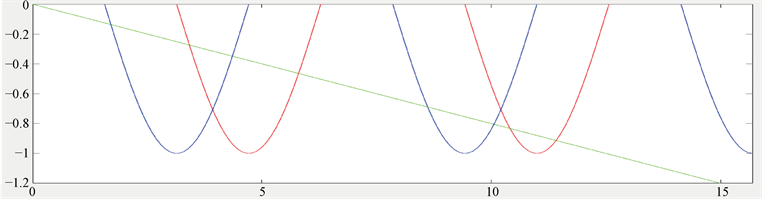Figure 2. The intersection of curve $v=\mathrm{sin}u$ and straight line $v=\frac{{k}_{7}}{{k}_{1}}u$

$\mathrm{cos}{u}_{1}<\mathrm{cos}{u}_{3}<\cdots <\mathrm{cos}{u}_{2⌊\frac{N}{2}⌋+1}<0<\mathrm{cos}{u}_{2⌊\frac{N}{2}⌋}<\cdots <\mathrm{cos}{u}_{2}<\mathrm{cos}0=1.$

$\left({u}_{1},{u}_{3},\cdots ,{u}_{2⌊\frac{N}{2}⌋+1},{u}_{2⌊\frac{N}{2}⌋},\cdots ,{u}_{2},0\right)=\left({w}_{1},{w}_{2},\cdots ,{w}_{N},{w}_{N+1}\right).$

$\mathrm{cos}{w}_{1}<\mathrm{cos}{w}_{2}<\cdots <\mathrm{cos}{w}_{N}<\mathrm{cos}{w}_{N+1}=1.$

${F}_{3}\left({E}_{0}\right)=-\left(g-{k}_{3}\right){x}_{0}^{2}+\left({k}_{2}\mathrm{cos}{u}_{0}+g-{k}_{4}\right).$

$f\left({w}_{1}\right)>f\left({w}_{2}\right)>\cdots >f\left({w}_{N}\right)>f\left({w}_{N+1}\right)={k}_{2}+g-{k}_{4}\text{.}$

(a) 如果 $f\left({w}_{1}\right)=f\left({u}_{1}\right)<0$，则系统(1)没有平衡点；

(b) 如果存在 $i\in \left\{1,2,\cdots ,N\right\}$，使得 $f\left({w}_{i}\right)>0>f\left({w}_{i+1}\right)$，则系统(1)有4i个平衡点

${P}_{±}^{\left(n\right)}=\left(±{x}_{n},±{x}_{n},\frac{±{x}_{n}+{k}_{7}{w}_{n}}{±{x}_{n}},{w}_{n}\right),n=1,2,\cdots ,i,$

${Q}_{±}^{\left(n\right)}=\left(±{x}_{n},±{x}_{n},\frac{±{x}_{n}-{k}_{7}{w}_{n}}{±{x}_{n}},-{w}_{n}\right),n=1,2,\cdots ,i,$

(c) 如果 $f\left({w}_{N+1}\right)=f\left(0\right)={k}_{2}+g-{k}_{4}>0$，则系统(1)有 $4N+2$ 个平衡点 ${P}_{±}^{\left(n\right)},{Q}_{±}^{\left(n\right)}\left(n=1,2,\cdots ,N\right)$

${E}_{±}=\left(±{x}_{N+1},±{x}_{N+1},1,0\right),$

${x}_{N+1}=\sqrt{\frac{{k}_{2}+g-{k}_{4}}{g-{k}_{3}}}\text{.}$

(II) ${k}_{5}>0$ 并且 $g-{k}_{3}>0$ 的情形

(1) 当 ${k}_{1}=0$${k}_{2}{k}_{7}\ne 0$ 并且 $-{k}_{5}+{k}_{2}+g-{k}_{4}\le 0$，可以得到 ${u}_{0}=0$ 以及 ${x}_{0}\left({z}_{0}-1\right)=0$。假设 ${x}_{0}\ne 0$，则 ${z}_{0}=1$ 并且有

${F}_{3}\left({E}_{0}\right)=-\left(g-{k}_{3}\right){x}_{0}^{2}+\left(-{k}_{5}{z}_{0}+{k}_{2}+g-{k}_{4}\right)<0.$

${E}_{0}\left(0,0,\frac{{k}_{2}+g-{k}_{4}}{{k}_{5}},0\right).$

(2) 当 ${k}_{1}{k}_{2}\ne 0$${k}_{7}=0$ 并且 $-{k}_{2}+g-{k}_{4}\le 0$ 时，可以得到 ${u}_{0}=k\pi$，于是 $\mathrm{cos}{u}_{0}=±1$。由 $-{k}_{2}+g-{k}_{4}\le 0$${k}_{2}\le 0$ 可得 $-{k}_{5}+{k}_{2}\mathrm{cos}{u}_{0}+g-{k}_{4}\le 0$。同样地，若假设 ${x}_{0}\ne 0$ 则有 ${z}_{0}=1$，并有

${F}_{3}\left({E}_{0}\right)=-\left(g-{k}_{3}\right){x}_{0}^{2}+\left(-{k}_{5}{z}_{0}+{k}_{2}\mathrm{cos}{u}_{0}+g-{k}_{4}\right)<0.$

${E}_{1,k}\left(0,0,\frac{{k}_{2}+g-{k}_{4}}{{k}_{5}},2k\pi \right),k\in ℤ,$

${E}_{2,k}\left(0,0,\frac{-{k}_{2}+g-{k}_{4}}{{k}_{5}},\left(2k+1\right)\pi \right),k\in ℤ\text{.}$

(3) 当 ${k}_{1}{k}_{7}\ne 0$${k}_{2}=0$ 并且 $g-{k}_{4}\le {k}_{5}$ 时，仍设 ${k}_{7}{u}_{0}={k}_{1}\mathrm{sin}{u}_{0}$ 的解为 $0,±{u}_{1},±{u}_{2},\cdots ,±{u}_{N}$。对于 ${u}_{0}=0$ 可以得到一个平衡点

${E}_{0}\left(0,0,\frac{g-{k}_{4}}{{k}_{5}},0\right).$

$\left(g-{k}_{3}\right){\left({k}_{7}{u}_{0}\right)}^{2}+{k}_{5}{z}_{0}{\left({z}_{0}-1\right)}^{2}=\left(g-{k}_{4}\right){\left({z}_{0}-1\right)}^{2}.$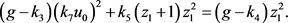(3)

${z}_{1}^{3}+\frac{\left[{k}_{5}-\left(g-{k}_{4}\right)\right]}{{k}_{5}}{z}_{1}^{2}+\frac{\left(g-{k}_{3}\right){\left({k}_{7}{u}_{0}\right)}^{2}}{{k}_{5}}=0.$ (4)

$\Delta =\frac{\left(g-{k}_{3}\right){\left({k}_{7}{u}_{0}\right)}^{2}}{{k}_{5}}\left({\left(\frac{\left[{k}_{5}-\left(g-{k}_{4}\right)\right]}{3{k}_{5}}\right)}^{3}+\frac{1}{4}\frac{\left(g-{k}_{3}\right){\left({k}_{7}{u}_{0}\right)}^{2}}{{k}_{5}}\right)>0.$

${E}_{0}=\left(0,0,\frac{g-{k}_{4}}{{k}_{5}},0\right),$

${P}_{±}^{\left(n\right)}=\left(\frac{{k}_{7}{u}_{n}}{z\left({u}_{n}\right)-1},\frac{{k}_{7}{u}_{n}}{z\left({u}_{n}\right)-1},z\left({u}_{n}\right),{u}_{n}\right),n=1,2,\cdots ,N,$

${Q}_{±}^{\left(n\right)}=\left(\frac{-{k}_{7}{u}_{n}}{z\left({u}_{n}\right)-1},\frac{-{k}_{7}{u}_{n}}{z\left({u}_{n}\right)-1},z\left({u}_{n}\right),-{u}_{n}\right),n=1,2,\cdots ,N,$

$\left(g-{k}_{3}\right){\left({k}_{7}{u}_{n}\right)}^{2}+{k}_{5}{z}_{0}{\left({z}_{0}-1\right)}^{2}=\left(g-{k}_{4}\right){\left({z}_{0}-1\right)}^{2}.$

2.3. 稳定性

$J=\left(\begin{array}{cccc}-r& r& 0& 0\\ m+z& -\left(1+m\right)& x& -{k}_{7}\\ g\left(2mx-\left(1+m\right)y\right)+2{k}_{3}x& -g\left(1+m\right)x& -{k}_{5}& -{k}_{2}\mathrm{sin}u\\ -1+z& 0& x& -{k}_{1}\mathrm{cos}u\end{array}\right).$

$C=g{x}_{0}^{2}\left(m+1\right),\text{\hspace{0.17em}}\text{\hspace{0.17em}}D=2r\left(g-{k}_{3}\right){x}_{0}^{2},\text{\hspace{0.17em}}\text{\hspace{0.17em}}M=m+1.$ (5)

(I) ${k}_{5}=0$ 的情形

(1) 当 ${k}_{1}=0$${k}_{7}\ne 0$ 并且 $\left({k}_{2}+g-{k}_{4}\right)\left(g-{k}_{3}\right)>0$ 时， ${E}_{±}$ 的特征多项式都为

$\mathrm{det}\left(J\left({E}_{±}\right)-\lambda I\right)={\lambda }^{4}+\left(1+m+r\right){\lambda }^{3}+C{\lambda }^{2}+\left(D-{k}_{7}C\right)\lambda -{k}_{7}D.$

$\left\{\begin{array}{l}{\Delta }_{1}=1+m+r>0,\\ {\Delta }_{2}=|\begin{array}{cc}1+m+r& D-{k}_{7}C\\ 1& C\end{array}|>0,\\ {\Delta }_{3}=|\begin{array}{ccc}1+m+r& D-{k}_{7}C& 0\\ 1& C& -{k}_{7}D\\ 0& 1+m+r& D-{k}_{7}C\end{array}|>0.\\ -{k}_{7}D>0.\end{array}$

$\left\{\begin{array}{l}\left(D-{k}_{7}C\right)\left[\left(M+r\right)C-\left(D-{k}_{7}C\right)\right]+{k}_{7}D{\left(M+r\right)}^{2}>0,\\ {k}_{7}<0

(2) 当 ${k}_{1}{k}_{2}\ne 0$${k}_{7}=0$ 并且 $|{k}_{4}-g|\le |{k}_{2}|$${E}_{±,k}$ 的特征方程皆为

$\mathrm{det}\left(J\left({E}_{±,k}\right)-\lambda I\right)=\left(\lambda +{k}_{1}\mathrm{sgn}\left({k}_{2}\left(g-{k}_{3}\right)\right)\right)\left({\lambda }^{3}+\left(1+m+r\right){\lambda }^{2}+C\lambda +D\right)\text{.}$

$\left\{\begin{array}{l}{k}_{1}{k}_{2}\left(g-{k}_{3}\right)>0,\\ {\Delta }_{1}=1+m+r>0,\\ {\Delta }_{2}=|\begin{array}{cc}1+m+r& D\\ 1& C\end{array}|>0,\\ D>0.\end{array}$

$\left\{\begin{array}{l}{k}_{1}{k}_{2}>0,\\ \left(M+r\right)gM-2r\left(g-{k}_{3}\right)>0,\\ g-{k}_{3}>0.\end{array}$

(II) ${k}_{5}>0$ 并且 $g-{k}_{3}>0$ 的情形

(1) 当 ${k}_{1}=0$${k}_{2}{k}_{7}\ne 0$ 并且 $-{k}_{5}-{k}_{2}+g-{k}_{4}\le 0$ 时， ${E}_{0}$ 的特征多项式为

$\mathrm{det}\left(J\left({E}_{0}\right)-\lambda I\right)=\left(\lambda +{k}_{5}\right)\left({\lambda }^{3}+\left(1+m+r\right){\lambda }^{2}-r\left({z}_{0}-1\right)\lambda +{k}_{7}r\left({z}_{0}-1\right)\right)\text{.}$

$\left\{\begin{array}{l}{\Delta }_{1}=1+m+r>0,\\ {\Delta }_{2}=|\begin{array}{cc}1+m+r& {k}_{7}r\left({z}_{0}-1\right)\\ 1& -r\left({z}_{0}-1\right)\end{array}|>0,\\ {k}_{7}r\left({z}_{0}-1\right)>0.\end{array}$

$\left\{\begin{array}{l}1+m+r+{k}_{7}>0,\\ {k}_{7}<0.\end{array}$

(2) 当 ${k}_{1}{k}_{2}\ne 0$${k}_{7}=0$ 并且 $-{k}_{2}+g-{k}_{4}\le 0$${E}_{1,k}$${E}_{2,k}$ 的特征多项式表示为

$\mathrm{det}\left(J\left({E}_{i,k}\right)-\lambda I\right)=\left(\lambda +{k}_{1}\mathrm{cos}{u}_{0}\right)\left(\lambda +{k}_{5}\right)\left({\lambda }^{2}+\left(1+m+r\right)\lambda -r\left({z}_{0}-1\right)\right)\text{.}$

${\lambda }_{3,4}=-\frac{1+m+r}{2}±\frac{1}{2}\sqrt{{\left(1+m+r\right)}^{2}+4r\left({z}_{0}-1\right)}\text{.}$

${z|}_{{E}_{1,k}}=\frac{{k}_{2}+g-{k}_{4}}{{k}_{5}}<{z|}_{{E}_{2,k}}=\frac{-{k}_{2}+g-{k}_{4}}{{k}_{5}}\le 0,$

${\left(\mathrm{cos}u\right)|}_{{E}_{1,k}}=1,\text{\hspace{0.17em}}\text{\hspace{0.17em}}\text{\hspace{0.17em}}{\left(\mathrm{cos}u\right)|}_{{E}_{2,k}}=-1,$

3. 混沌性质

3.1. 没有平衡点的混沌系统

$\stackrel{\to }{p}=\left(0.01,0.1,10,0,-100,9,-10,0,-1\right)$，此时系统(1)没有平衡点。选取初始点为 $\left(1,1,1,1\right)$ 可得系统(1)是混沌的，四个李雅普诺夫指数为

$\left({\lambda }_{LE1},{\lambda }_{LE2},{\lambda }_{LE3},{\lambda }_{LE4}\right)=\left(1.0730,0.0011,-0.5052,-1.6793\right),$

3.2. 具有有限个平衡点的超混沌/混沌系统

3.2.1. 具有两个平衡点的超混沌系统

$\stackrel{\to }{p}=\left(0.1,1,0.01,-1,-0.1,-1,-10,0,-1\right)$，此时系统(1)有两个非双曲平衡点

${E}_{±}=\left(±3.1324,±3.1324,1,0\right)\text{.}$

$\left({\lambda }_{LE1},{\lambda }_{LE2},{\lambda }_{LE3},{\lambda }_{LE4}\right)=\left(0.6009,0.6005,-0.0002,-2.3013\right),$Figure 3. The parameter group is $\stackrel{\to }{p}=\left(0.01,0.1,10,0,-100,9,-10,0,-1\right)$, when the initial condition is selected as $\left(1,1,1,1\right)$, the system (1 ) x-y-z space phase diagram (left picture) and Poincaré mapping on xz plane (right picture)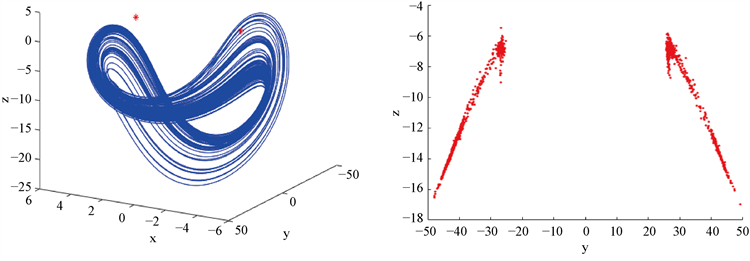Figure 4. When the parameter group is $\stackrel{\to }{p}=\left(0.1,1,0.01,-1,-0.1,-1,-10,0,-1\right)$, when the initial conditions are selected as $\left(3.1334,3.1334,1.0010,0.0010\right)$, the system (1) the x-y-z space phase diagram (left picture) and the Poincaré map on the yz plane (right picture)

3.2.2. 具有六个平衡点的混沌系统

$\stackrel{\to }{p}=\left(10,0.1,1,-1,0,0,-1,0,-0.8\right)$，此时系统(1)有六个不稳定的平衡点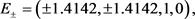${P}_{+}^{\left(1\right)}=\left(1.4142,1.4142,0.3602,1.1311\right),$

${P}_{-}^{\left(1\right)}=\left(-1.4142,-1.4142,1.6398,1.1311\right),$

${Q}_{+}^{\left(1\right)}=\left(1.4142,1.4142,1.6398,-1.1311\right),$

${Q}_{-}^{\left(1\right)}=\left(-1.4142,-1.4142,0.3602,-1.1311\right),$

$\left({\lambda }_{LE1},{\lambda }_{LE2},{\lambda }_{LE3},{\lambda }_{LE4}\right)=\left(0.1475,0.0009,-0.6082,-10.4245\right)$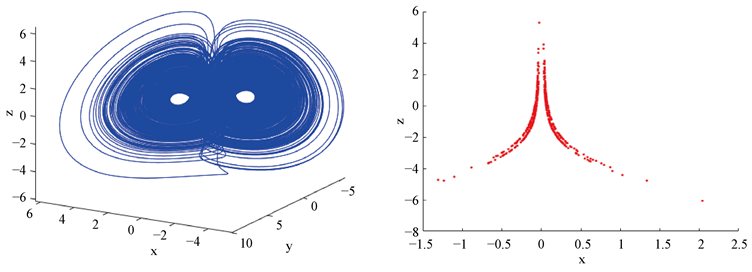Figure 5. When the parameter group is $\stackrel{\to }{p}=\left(10,0.1,1,-1,0,0,-1,0,-0.8\right)$, when the initial conditions are selected as $\left(1.4152,1.4152,1.0010,0.0010\right)$ the system (1) x-y-z space phase diagram (left picture) and Poincaré mapping on x-z plane (right picture)

3.3. 具有无穷多个平衡点的混沌系统

3.3.1. 具有无穷多个孤立平衡点的混沌系统

$\stackrel{\to }{p}=\left(10,0.1,1,-1,-1,0,0,0.1,0\right)$，此时系统(1)有无穷多个不稳定的孤立平衡点

${E}_{1,k}=\left(0,0,0,2k\pi \right),k\in ℤ,$

${E}_{2,k}=\left(0,0,20,\left(2k+1\right)\pi \right),k\in ℤ\text{.}$

$\left({\lambda }_{LE1},{\lambda }_{LE2},{\lambda }_{LE3},{\lambda }_{LE4}\right)=\left(0.1126,0.0001,-0.8714,-11.3100\right)$

3.3.2. 具有一条线平衡点的混沌系统

$\stackrel{\to }{p}=\left(0.01,0.1,90,0,-100,9,-10,0,-1\right)$，此时系统(1)有一条直线平衡点

${E}^{\text{*}}\left(0,0,{z}^{*},0\right),{z}^{*}\in ℝ\text{.}$

$\left({\lambda }_{LE1},{\lambda }_{LE2},{\lambda }_{LE3},{\lambda }_{LE4}\right)=\left(0.0163,-0.0003,-0.0277,-1.0982\right)\text{.}$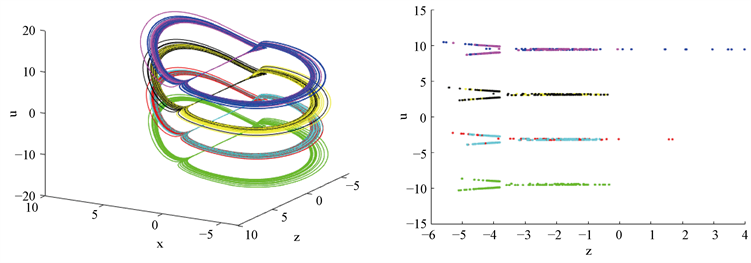Figure 6. When the parameter group is $\stackrel{\to }{p}=\left(10,0.1,1,-1,-1,0,0,0.1,0\right)$ and the initial condition is selected as $\left(0.001,0.001,0.001,0.001\right)$, the system (1) the x-y-u space phase diagram (left picture) and the Poincaré map on the z-u plane (right picture)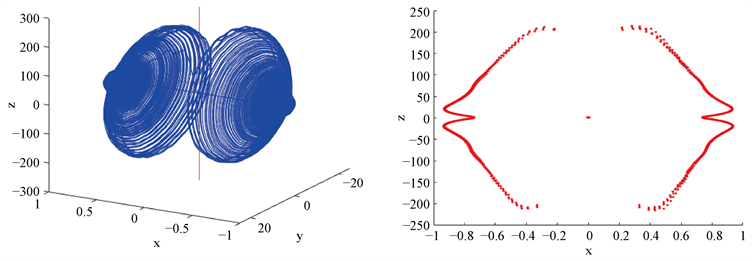Figure 7. The parameter group is $\stackrel{\to }{p}=\left(0.01,0.1,90,0,-100,9,-10,0,-1\right)$, when the initial condition is selected as $\left(0.001,0.001,0.001,0.001\right)$, the system (1) x-y-z space phase diagram (left picture) and Poincaré mapping on x-z plane (right picture)

4. Hopf分叉

4.1. Hopf分叉的存在性和周期轨的稳定性

${k}_{1}^{2}+{k}_{2}^{2}+{k}_{3}^{2}+{k}_{5}^{2}=0$${k}_{7}\ne 0$ 并且 $g-{k}_{4}>0$ 时，系统(1)有两个平衡点 ${O}_{±}\left(±{x}_{0},±{x}_{0},1,0\right)$，其中 ${x}_{0}=\sqrt{\left(g-{k}_{4}\right)/g}$

$S=\left\{\left(r,m,g,{k}_{1},{k}_{2},{k}_{3},{k}_{4},{k}_{5},{k}_{7}\right)|\begin{array}{c}{k}_{1}^{2}+{k}_{2}^{2}+{k}_{3}^{2}+{k}_{5}^{2}=0,r,m,g>0,{k}_{7}<0,\\ \left(1+m+r+4{k}_{7}\right)\left(1+m\right)-4r\ge 0,{k}_{4}={k}_{4}^{0}\end{array}\right\},$

${k}_{4}^{0}=g-\frac{2r{k}_{7}{\left(1+m+r\right)}^{2}}{A\left[A-\left(1+m\right)\left(1+m+r\right)\right]},$

$A=2r-{k}_{7}\left(1+m\right).$

$\stackrel{\to }{p}\in S\cap {S}_{0}$ 时，系统(1)有两个平衡点 ${E}_{±}$，并且它们都在 ${k}_{4}={k}_{4}^{0}$ 处经历了Hopf分叉。

$A-\left(1+m\right)\left(1+m+r\right)=2r-\left(1+m+r+{k}_{7}\right)\left(1+m\right)<4r-\left(1+m+r+4{k}_{7}\right)\left(1+m\right)\le 0$

$A=2r-{k}_{7}\left(1+m\right)>0.$

$g-{k}_{4}=\frac{2r{k}_{7}{\left(1+m+r\right)}^{2}}{A\left[A-\left(1+m\right)\left(1+m+r\right)\right]}>0.$

${O}_{±}$ 的特征方程为

$f\left(\lambda \right)={\lambda }^{4}+\left(1+m+r\right){\lambda }^{3}+C{\lambda }^{2}+\left(D-{k}_{7}C\right)\lambda -{k}_{7}D,$ (6)

${\omega }^{2}=\frac{D-{k}_{7}C}{1+m+r}>0$ (7)

${\left(D-{k}_{7}C\right)}^{2}-C\left(D-{k}_{7}C\right)\left(1+m+r\right)-{k}_{7}D{\left(1+m+r\right)}^{2}=0.$

$A\left(g-{k}_{4}\right)\left[A-\left(1+m\right)\left(1+m+r\right)\right]=2r{k}_{7}{\left(1+m+r\right)}^{2},$

$g-{k}_{4}=\frac{2r{k}_{7}{\left(1+m+r\right)}^{2}}{A\left[A-\left(1+m\right)\left(1+m+r\right)\right]},$

$f\left(\lambda \right)=\left({\lambda }^{2}+{\omega }^{2}\right)\left({\lambda }^{2}+E\lambda +F\right)={\lambda }^{4}+E{\lambda }^{3}+\left(F+{\omega }^{2}\right){\lambda }^{2}+{\omega }^{2}E\lambda +{\omega }^{2}F,$ (8)

$E=1+m+r>0,$ (9)

$F=C-{\omega }^{2}=C-\frac{D-{k}_{7}C}{1+m+r}=\frac{g-{k}_{4}}{1+m+r}\left[\left(1+m\right)\left(1+m+r\right)-A\right]=\frac{-2r{k}_{7}\left(1+m+r\right)}{A}>0.$

$\Delta ={E}^{2}-4F=\frac{\left(1+m+r\right)}{A}\left[A\left(1+m+r\right)+8r{k}_{7}\right]\ge \frac{2r\left(1+m+r\right)}{A}\left(1+m+r+4{k}_{7}\right)>0.$ (10)

$\frac{\text{d}\lambda }{\text{d}{k}_{4}}=\frac{\left(1+m\right){\lambda }^{2}+\left[2r-{k}_{7}\left(1+m\right)\right]\lambda }{4{\lambda }^{3}+3\left(1+m+r\right){\lambda }^{2}+2C\lambda +\left(D-{k}_{7}C\right)}\text{.}$

$\lambda =i\omega$ 并且观察到式(7)，于是

${\frac{\text{d}\lambda }{\text{d}{k}_{4}}|}_{{k}_{4}={k}_{4}^{0}}=\frac{-\left(1+m\right){\omega }^{2}+\left[2r-{k}_{7}\left(1+m\right)\right]i\omega }{-4i{\omega }^{3}-3\left(1+m+r\right){\omega }^{2}+2Ci\omega +\left(1+m+r\right){\omega }^{2}}=\frac{\left(1+m\right)\omega -\left[2r-{k}_{7}\left(1+m\right)\right]i}{4i{\omega }^{2}+2\left(1+m+r\right)\omega -2Ci}\text{.}$

$\begin{array}{c}\mathrm{sgn}\left[\mathrm{Re}\left({\frac{\text{d}\lambda }{\text{d}{k}_{4}}|}_{{k}_{4}={k}_{4}^{0}}\right)\right]=\mathrm{sgn}\left[\left(1+m\right)\left(1+m+r\right){\omega }^{2}-\left[2r-{k}_{7}\left(1+m\right)\right]\left(2{\omega }^{2}-C\right)\right]\\ =\mathrm{sgn}\left\{\left[\left(1+m+r+2{k}_{7}\right)\left(1+m\right)-4r\right]{\omega }^{2}+\left[2r-{k}_{7}\left(1+m\right)\right]C\right\}=1,\end{array}$

$\mathrm{Re}\left({\frac{\text{d}\lambda }{\text{d}{k}_{4}}|}_{{k}_{4}={k}_{4}^{0}}\right)>0.$ (11)

${l}_{1}=\frac{g{r}^{3}{\omega }^{3}\left\{2{M}^{2}{\omega }^{4}\left[2M{\omega }^{2}+5{k}_{7}\left(M{k}_{7}+2r\right)\right]+r{k}_{7}b\right\}}{\left(g-{k}_{4}\right)\left[{\left(M{\omega }^{2}+4r{k}_{7}\right)}^{2}+{A}^{2}{\omega }^{2}\right]\left[{\left(5r{k}_{7}+2M{\omega }^{2}\right)}^{2}+{A}^{2}{\omega }^{2}\right]},$

$A=2r-M{k}_{7},$

$b=\left(64{M}^{2}{k}_{7}^{2}+5rM{k}_{7}-4{r}^{2}\right){\omega }^{2}+35r{k}_{7}^{2}\left(3M{k}_{7}-2r\right),$

$M=m+1.$

(I) 如果 ${l}_{1}\ne 0$，则系统(1)将同时在平衡点 ${O}_{±}$ 处发生Hopf分叉，并且在 ${k}_{4}={k}_{4}^{0}$ 附近产生的两个分叉周期轨道在 ${l}_{1}<0$ 时是稳定的，在 ${l}_{1}>0$ 时是不稳定的。

${X}^{2}={x}_{0}^{2}=\frac{g-{k}_{4}}{g}\text{.}$ (12)

$\left\{\begin{array}{l}{x}_{1}=x-X,\\ {y}_{1}=y-X,\\ {z}_{1}=z-1,\\ {u}_{1}=u,\end{array}$

$\left\{\begin{array}{l}\stackrel{˙}{x}=r\left(y-x\right),\\ \stackrel{˙}{y}=M\left(x-y\right)+\left(x+X\right)z-{k}_{7}u,\\ \stackrel{˙}{z}=g\left[\left(M-1\right){x}^{2}+1-Mxy+\left(M-2\right)Xx-MXy-{X}^{2}\right]-{k}_{4},\\ \stackrel{˙}{u}=\left(x+X\right)z.\end{array}$

$A=\left(\begin{array}{cccc}-r& r& 0& 0\\ M& -M& X& -{k}_{7}\\ g\left(M-2\right)X& -gMX& 0& 0\\ 0& 0& X& 0\end{array}\right),$

$p=\frac{1}{P}\left(\begin{array}{c}-M\left(gX{k}_{7}+{\omega }^{2}\right)+\left({\omega }^{2}-gXM\right)\omega i\\ -r{\omega }^{2}\\ -rX\left(\omega i+{k}_{7}\right)\\ {k}_{7}r\omega i\end{array}\right),$

$q=\left(\begin{array}{c}r\omega i\\ r\omega i-{\omega }^{2}\\ -gX\left(2r+M\omega i\right)\\ \frac{\left[\left(M+r\right){\omega }^{2}-2rg{X}^{2}\right]+\left({\omega }^{2}-g{X}^{2}M\right)\omega i}{{k}_{7}}\end{array}\right),$

$B\left(x,y\right)=\left(\begin{array}{c}0\\ {x}_{1}{y}_{3}+{x}_{3}{y}_{1}\\ g\left[2\left(M-1\right){x}_{1}{y}_{1}-M\left({x}_{1}{y}_{2}+{x}_{2}{y}_{1}\right)\right]\\ {x}_{1}{y}_{3}+{x}_{3}{y}_{1}\end{array}\right),$

$C\left(x,y,z\right)=\left(\begin{array}{c}0\\ 0\\ 0\\ 0\end{array}\right),$

$P=2rg{X}^{2}\left[\left(M{\omega }^{2}+4r{k}_{7}\right)+\left(2r-{k}_{7}M\right)\omega i\right]\text{.}$

${A}^{-1}B\left(q,\stackrel{¯}{q}\right)=\frac{{r}^{2}{\omega }^{2}}{X}\left(\begin{array}{c}1\\ 1\\ \frac{-2gXM}{r}\\ 0\end{array}\right),$

${\left(2\omega iE-A\right)}^{-1}B\left(q,q\right)=-2rgX\omega iR\left(\begin{array}{c}r\left(2\omega i-{k}_{7}\right)\\ \left(2\omega i-{k}_{7}\right)\left(2\omega i+r\right)\\ \frac{1}{X}\left[-4{\omega }^{2}\left(M+2\omega i+r\right)-\frac{H}{R}\right]\\ 2\omega i\left(M+2\omega i+r\right)\end{array}\right),$

$H=2r+\left(m+1\right)\omega i,$

$R=\frac{5r\omega i-3M{\omega }^{2}}{6g{X}^{2}\left[\left(5r{k}_{7}+2M{\omega }^{2}\right)-\left(2r-{k}_{7}M\right)\omega i\right]}.$

${l}_{1}=\frac{{r}^{3}{\omega }^{3}\left\{2{M}^{2}{\omega }^{4}\left[2M{\omega }^{2}+5{k}_{7}\left(M{k}_{7}+2r\right)\right]+r{k}_{7}b\right\}}{{X}^{2}\left[{\left(M{\omega }^{2}+4r{k}_{7}\right)}^{2}+{a}^{2}{\omega }^{2}\right]\left[{\left(5r{k}_{7}+2M{\omega }^{2}\right)}^{2}+{a}^{2}{\omega }^{2}\right]},$

$a=2r-M{k}_{7},$

$b=\left(64{M}^{2}{k}_{7}^{2}+5rM{k}_{7}-4{r}^{2}\right){\omega }^{2}+35r{k}_{7}^{2}\left(3M{k}_{7}-2r\right),$

$M=m+1.$

${l}_{1}=\frac{g{r}^{3}{\omega }^{3}\left\{2{M}^{2}{\omega }^{4}\left[2M{\omega }^{2}+5{k}_{7}\left(M{k}_{7}+2r\right)\right]+r{k}_{7}b\right\}}{\left(g-{k}_{4}\right)\left[{\left(M{\omega }^{2}+4r{k}_{7}\right)}^{2}+{a}^{2}{\omega }^{2}\right]\left[{\left(5r{k}_{7}+2M{\omega }^{2}\right)}^{2}+{a}^{2}{\omega }^{2}\right]}\text{.}$

4.2. 数值模拟

$\left(r,m,g,{k}_{1},{k}_{2},{k}_{3},{k}_{5},{k}_{7}\right)=\left(0.1,1,0.01,0,0,0,0,-0.1\right),$Figure 8. The parameter group is $\stackrel{\to }{p}=\left(0.1,1,0.01,0,0,0,-0.0480,0,-0.1\right)$ select initial conditions $\left(±2.4099,±2.4099,1.0010,0.0010\right)$, system (1) there are two unstable limit cycles

5. 总结

 Leonov, G.A., Kuznetsov, N.V. and Mokaev, T.N. (2015) Homoclinic Orbits, and Self-Excited and Hidden Attractors in a Lorenz-Like System Describing Convective Fluid Motion. The European Physical Journal: Special Topics, 224, 1421-1458.
https://doi.org/10.1140/epjst/e2015-02470-3

 Ojoniyi, O.S. and Njah, A.N. (2016) A 5D Hyperchaotic Sprott B System with Coexisting Hidden Attractors. Chaos, Solitons and Fractals: Applications in Science and Engineering: An Interdisciplinary Journal of Nonlinear Science, 87, 172-181.
https://doi.org/10.1016/j.chaos.2016.04.004

 Viet-Thanh, P., Jafari, S., Kapitaniak, T., et al. (2017) Generating a Chaotic System with One Stable Equilibrium. International Journal of Bifurcation and Chaos in Applied Sciences and Engineering, 27, Article ID: 1750053.
https://doi.org/10.1142/S0218127417500535

 Singh, J.P., Roy, B.K. and Jafari, S. (2018) New Family of 4-D Hyperchaotic and Chaotic Systems with Quadric Surfaces of Equilibria. Chaos, Solitons and Fractals: Applications in Science and Engineering: An Interdisciplinary Journal of Nonlinear Science, 106, 243-257.
https://doi.org/10.1016/j.chaos.2017.11.030

 Yang, T. (2020) Multistability and Hidden Attractors in a Three-Dimensional Chaotic System. International Journal of Bifurcation and Chaos, 30, Article ID: 2050087.
https://doi.org/10.1142/S021812742050087X

 Rajagopal, K., Khalaf, A.J.M., Wei, Z.C., et al. (2019) Hyperchaos and Coexisting Attractors in a Modified van der Pol-Duffing Oscillator. International Journal of Bifurcation and Chaos, 29, Article ID: 1950067.
https://doi.org/10.1142/S0218127419500676

 Lorenz, E.N. (1963) Deterministic Nonperiodic Flow. Journal of the Atmospheric Sciences, 20, 130-141.
https://doi.org/10.1175/1520-0469(1963)020<0130:DNF>2.0.CO;2

 Chen, G.R. and Ueta, T. (1999) Yet An-other Chaotic Attractor. International Journal of Bifurcation and Chaos in Applied Sciences and Engineering, 9, 1465-1466.
https://doi.org/10.1142/S0218127499001024

 Chen, Y.M. and Yang, Q.G. (2013) The Nonequiv-alence and Dimension Formula for Attractors of Lorenz-Type Systems. International Journal of Bifurcation and Chaos in Applied Sciences and Engineering, 23, 1350200-1-1350200-12.
https://doi.org/10.1142/S0218127413502003

 Cang, S.J., Li, Y., Zhang, R.Y., et al. (2019) Hidden and Self-Excited Coexisting Attractors in a Lorenz-Like System with Two Equilibrium Points. Nonlinear Dynamics, 95, 381-390.
https://doi.org/10.1007/s11071-018-4570-x

 Lu, J.H. and Chen, G.R. (2002) A New Chaotic At-tractor Coined. International Journal of Bifurcation and Chaos in Applied Sciences and Engineering, 12, 659-661.
https://doi.org/10.1142/S0218127402004620

 Rössler, O.E. (1976) An Equation for Continuous Chaos. Physics Letters A, 57, 397-398.
https://doi.org/10.1016/0375-9601(76)90101-8

 Tutueva, A., Tutueva, A., Butusov, D., et al. (2019) The Dynamical Analysis of the Modified Rossler System. IOP Conference Series: Materials Science and Engineering, 630, Article ID: 012006.
https://doi.org/10.1088/1757-899X/630/1/012006

 Wei, Z.C., Wang, R.R. and Liu, A.P. (2014) A New Find-ing of the Existence of Hidden Hyperchaotic Attractors with No Equilibria. Mathematics and Computers in Simulation, 100, 13-23.
https://doi.org/10.1016/j.matcom.2014.01.001

 Jafari, S. and Sprott, J.C. (2015) Simple Chaotic Flows with a Line Equilibrium (Vol. 57, pg 79, 2013). Chaos, Solitons and Fractals: Applications in Science and En-gineering: An Interdisciplinary Journal of Nonlinear Science, 77, 341-342.
https://doi.org/10.1016/j.chaos.2015.05.002

 Yang, Q.G. and Qiao, X.M. (2019) Constructing a New 3D Chaotic System with Any Number of Equilibria. International Journal of Bifurcation and Chaos, 29, Article ID: 1950060.
https://doi.org/10.1142/S0218127419500603

 Moffatt, H.K. (1979) A Self-Consistent Treatment of Simple Dynamo Systems. Geophysical & Astrophysical Fluid Dynamics, 14, 147-166.
https://doi.org/10.1080/03091927908244536

 Kuznetsov, Y.A. (1998) Elements of Applied Bifurcation Theory. Springer, New York.

Top